# Calculate the nuclear binding energy (in J) and the binding energy per nucleon for the following isotope: 129 Sb (128.9091 amu) 51 10 Nuclear binding energy 27 12 Binding energy per nucleon-2.1 ucleo...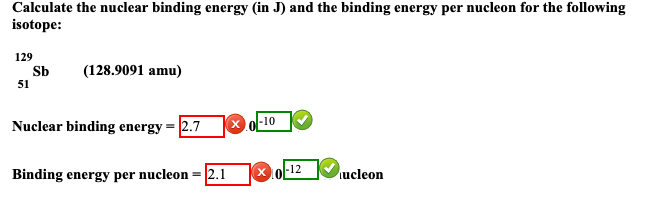Calculate the nuclear binding energy (in J) and the binding energy per nucleon for the following isotope: 129 Sb (128.9091 amu) 51 10 Nuclear binding energy 27 12 Binding energy per nucleon-2.1 ucleon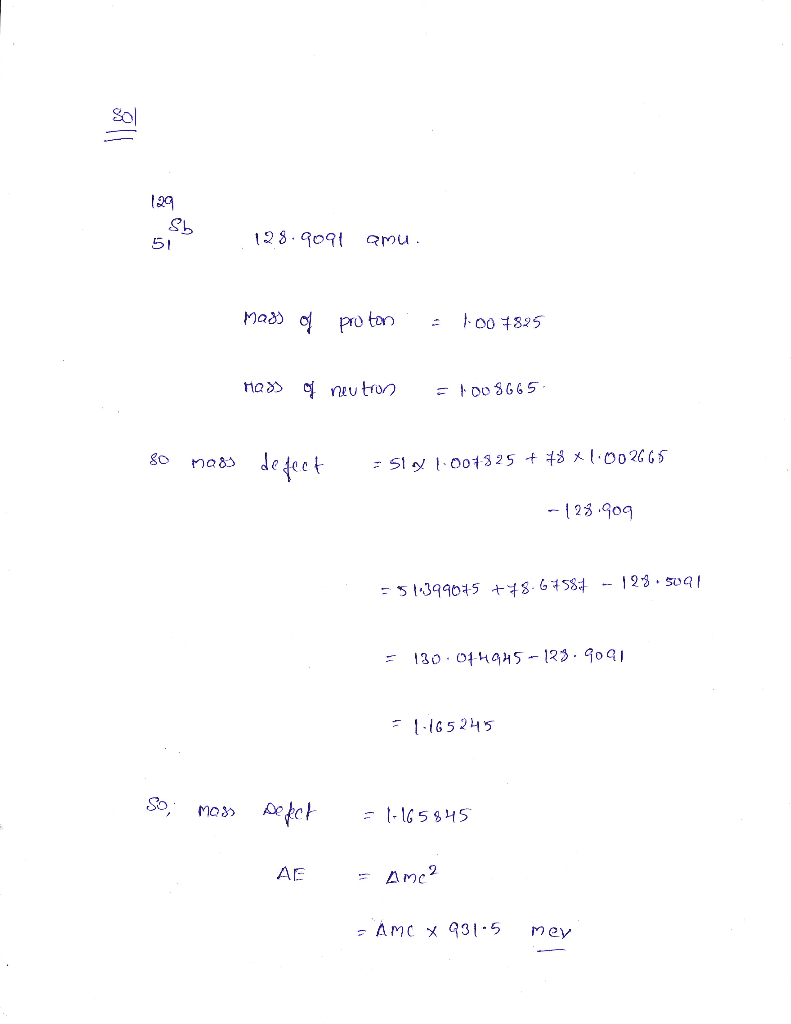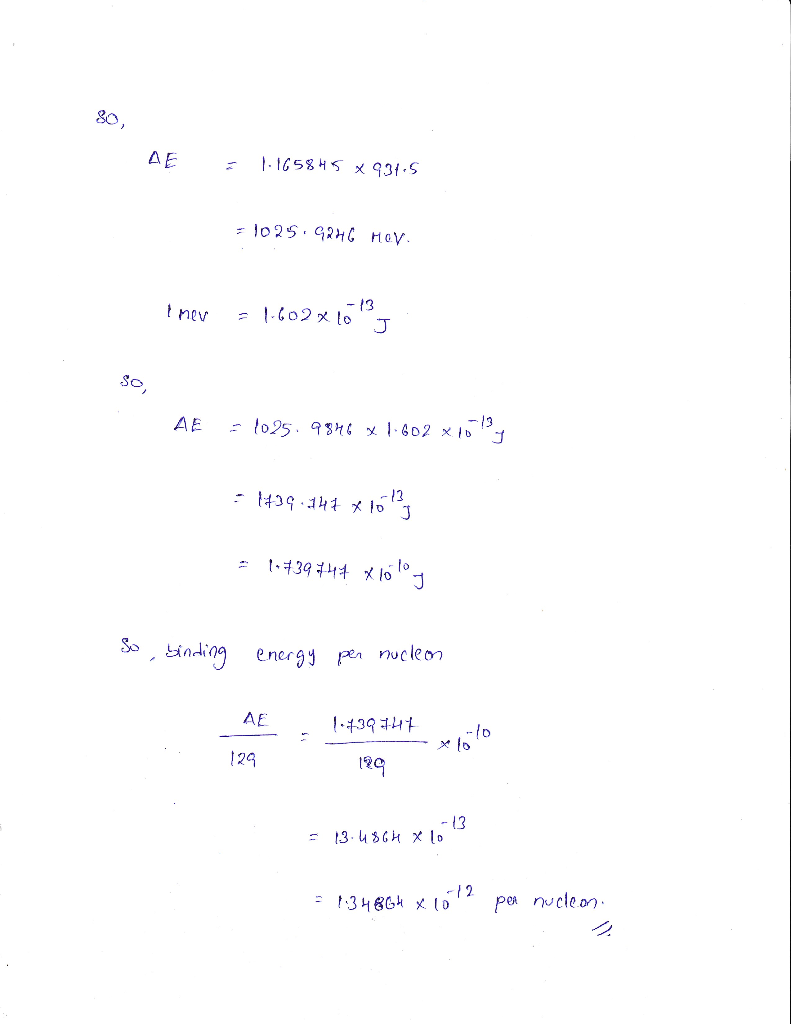##### Add Answer of: Calculate the nuclear binding energy (in J) and the binding energy per nucleon for the following isotope: 129 Sb (128.9091 amu) 51 10 Nuclear binding energy 27 12 Binding energy per nucleon-2.1 ucleo...
Similar Homework Help Questions
• ### Nuclear Binding energy / mass defect HELP!!!

Calculate the mass defect and nuclear binding energy per nucleon of the each of the nuclides indicated below.V-51 (atomic mass = 50.94396 amu)calculate the mass in amu (5 decimal places)Calculate the binding energy per nucleon in MeV/nucleon to four sig figs

• ### Incorrect Question 6 0/1 pts The isotope with the greatest nuclear binding energy per nucleon is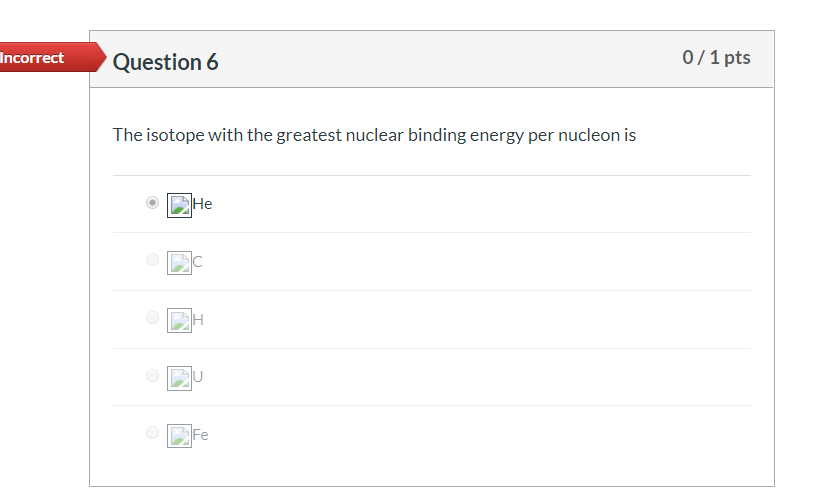Incorrect Question 6 0/1 pts The isotope with the greatest nuclear binding energy per nucleon is

• ### calculate nuclear binding energy

Calculate the nuclear binding energy 35Cl in the following: The actual mass of a chlorine-35 atom is 34.9689 amu.a) Kilojoules per mole of Cl atomsb) Kilojoules per gram of Cl atomsc) Megaelectron volts per nucleon

• ### Binding energy per nucleon

What is the binding energy per nucleon, in joules, of a chlorine-35 atom, given that the atomic mass equals 34.9595 amu?(e- mass = 0.00055 amu, n mass = 1.00867 amu, p mass = 1.00728 amu, c = 3.00 x 10^8 m/s, 1 amu = 1.6606 x 10^-24 g, 1 J = 1 kG m^2/s^2)a. 1.56 x 10^-11 J/atomb. 1.56 x 10^-12 J/atomc. 4.93 x 10^-11 J/atomd. 5.33 x 10^-11 J/atome. 1.56 x 10^-10 J/atom

• ### Find the nuclear binding energy

Find the nuclear binding energy of potassium-40 (atomic mass =39.9632591 amu) in units of joules per nucleon. [Data: neutron mass= 1.674928 x 10-24g;proton mass= 1.672623 x10-24g; electron mass = 9.109387 x 10-28g;NA = 6.0221367 x 1023/ mol; c= 2.99792458x108 m/s]A.1.37 x 10-12 J/nucleonB. 5.48 x 10-11 J/nucleonC. 5.64 x 10-11 J/nucleonD. 1.41 x 10-12 J/nucleonE. 2.97 x 10 -12 J/nucleon

• ### calculate the binding energy per nucleon for the following isotopes.

Calculate the binding energy per nucleon for the following isotopes.(a) 64Zn___ MeV/nucleon(b) 59Co___ MeV/nucleon(c) 55Mn___(d) 195Pt____ MeV/nucleon

• ### 2. Calculate the binding energy per nucleon in 24Mg if the atomic mass excess value is...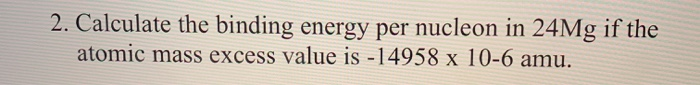2. Calculate the binding energy per nucleon in 24Mg if the atomic mass excess value is - 14958 x 10-6 amu.

• ### total binding energy and binding energy per nucleonCalculate the total binding energy, and the binding energy per nucleon (in MeV), for the following:and

• ### (6 points) Find the binding energy per nucleon in J and eV for the three following...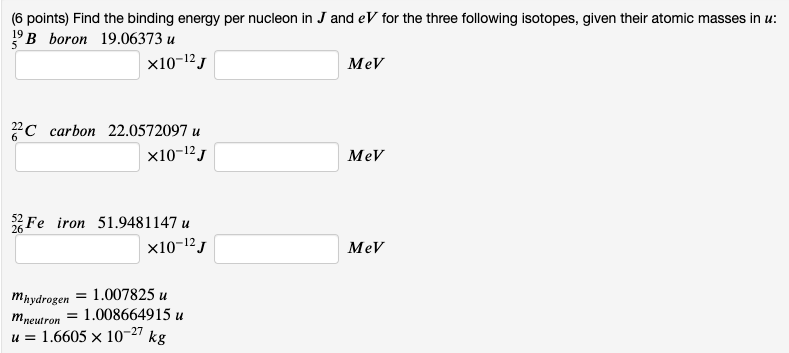(6 points) Find the binding energy per nucleon in J and eV for the three following isotopes, given their atomic masses in u B boron 19.06373 u x10-12 MeV 22C carbon 22.0572097 u x10-12, MeV 52 Fe iron 51.9481147 u x10-12) MeV mhydrogen = 1.007825 u mneutron = 1.008664915 u u = 1.6605 x 10-27 kg

• ### 17. The mass of the atom 1°F is 18.99840 amu. (a) Calculate its binding energy per...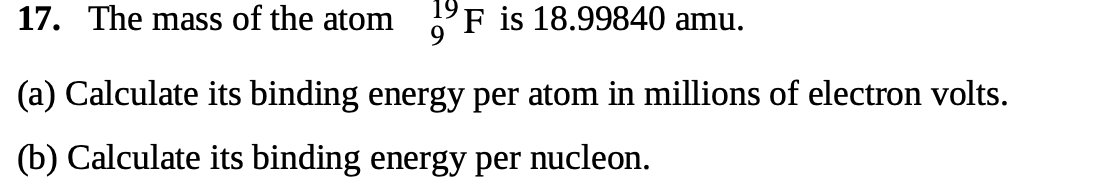17. The mass of the atom 1°F is 18.99840 amu. (a) Calculate its binding energy per atom in millions of electron volts. (b) Calculate its binding energy per nucleon.

Need Online Homework Help?